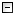Home Control Panel Login Register Search Submit Logout About me Contact meMy ResearchWeb This Site
 Home > Plot histogram in Excel - An easy way
Plot histogram in Excel - An easy way
Cite this page: (2005). Plot histogram in Excel - An easy way. Retrieved December 6, 2023, from https://www.psychstat.org/us/article.php/10.htm.
Plot histogram in Excel - An easy way
If you have a variable with a list of values like,
1
2
3
4
5
6
7
8
9
10
9
8
7
6
5
4
3
2
1
Maybe you want plot the histogram in Excel.  However, Excel doesn't plot the histogram for you automatically in plot menu.
One solution is to count the frequency first, then use the bar plot.
There is a trick to count the frequency. You need using the array method.
After you input the data, you need to input the formula like this
 =SUM(IF($B$2:$B$20<=2,1,0)) =SUM(IF($B$2:$B$20<=5,IF($B$2:$B$20>2,1,0),0)) 4 5 9

After you type in the formual, push down SHIFT+CTRL simultanuously and then PUSH down ENTER button. Then the frequency will be calculated.

Now you can plot!

Submitted by: johnny
 Add a comment View comment Add to my favorite Email to a friend Print1 2 3 4 5 6 7 8 9 10
 Copyright © 2003-13 Zhiyong Zhang \'s Psychometric WebsiteMaintained by Zhiyong Zhang